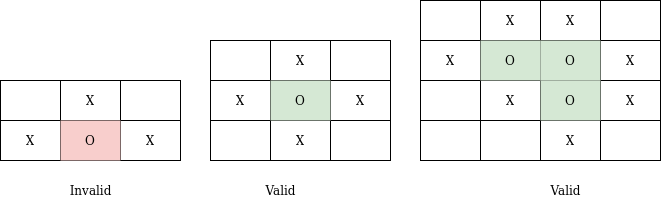# Capture region

Posted: 14 Jan, 2021
Difficulty: Moderate

## PROBLEM STATEMENT

#### You are given a matrix having ‘N’ rows and ‘M’ columns. Each cell of the matrix is either ‘X’ or ‘O’. You need to flip all those regions of ‘O’ which are surrounded by ‘X’ i.e. you need to change all ‘O’s which are present in the region to ‘X’.

##### Note
``````1. Surrounded regions shouldn’t be on the border, which means that any 'O' on the border of the matrix is not flipped to 'X'.
2. Any ‘O’ or group of connected ‘O’ are said to be surrounded by ‘X’ when all cells touching the boundary of the group of ‘O’ must contain ‘X’.
``````
##### For example##### Input Format:
``````The first line of the input contains an integer ‘T’ denoting the number of test cases.

The next 2 * T lines describe the ‘T’ test cases.

The first line of each test case contains two space-separated positive integers ‘N’ and ‘M’ denoting the number of the rows and columns in a matrix respectively.

In the next ‘N’ lines of each test case, the ith line contains a string of length ‘M’ containing either ‘X’ or ‘O’.
``````
##### Output Format:
``````For each test case, print N lines each line containing M characters denoting the matrix element at position MATRIX[i][j] where i and j are the indices of the 2 - D array.

The output of each test case will begin from a new line.
``````
##### Note:
``````You do not need to print anything, it has already been taken care of. Just implement the given function.
``````
##### Constraints:
``````1 <= T <= 50
1 <= N <= 10 ^ 4
1 <= M <= 10 ^ 4
1 <= N * M <= 10 ^ 4

Where ‘T’ is the number of test cases, ‘N’ is the number of rows, ‘M’ is the number of columns.

Time Limit: 1 sec
``````Approach 1
• We will run two nested loops to traverse each cell of the matrix.
• For each cell containing ‘O’, we perform DFS in which we go to all adjacent neighbors containing ‘O’.
• The DFS function will tell us whether from this cell we can reach the boundary or not. If there is a way to reach the boundary from any of the neighbor cells of the current cell then this current cell can also reach the boundary through the neighbor cell. Hence this cell can’t be part of a region.
• If there is no way to reach the boundary then the current cell will be changed to ‘X’.